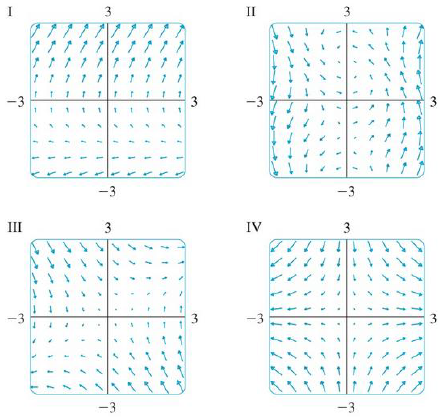Chapter 16.1, Problem 13E

Chapter
Section
Textbook Problem

Match the vector fields F with the plots labeled I-IV. Give reasons for your choices.13. F(x, y) = ⟨y, y + 2⟩To determine

To match: The vector field F(x,y)=y,y+2 with the plots labelled as I-IV.

Explanation

Given data:

F(x,y)=y,y+2

Formula used:

Consider a two-dimensional vector F=x,y .

Write the expression for length of the two dimensional vector.

|F(x,y)|=x2+y2 (1)

Find the length of F(x,y) using equation (1).

|F(x,y)|=(y)2+(y+2)2

Consider a certain interval of x as (2,2) and y as (2,2) to plot F(x,y) .

The estimated values of |F(x,y)| and F(x,y) for different values of x and y are shown in Table 1.

Table 1

 Quadrant (x,y) |F(x,y)|=(y)2+(y+2)2 F(x,y)=〈y,y+2〉 I (0,0) 2 〈0,2〉 (1,0) 2 〈0,2〉 (2,0) 2 〈0,2〉 (0,1) 3.162 〈1,3〉 (1,1) 3.162 〈1,3〉 (0,2) 4

Still sussing out bartleby?

Check out a sample textbook solution.

See a sample solution

The Solution to Your Study Problems

Bartleby provides explanations to thousands of textbook problems written by our experts, many with advanced degrees!

Get Started

In Exercises 35-42, find functions f and g such that h = g f. (Note: The answer is not unique.) 40. h(x)=1x24

Applied Calculus for the Managerial, Life, and Social Sciences: A Brief Approach

Solve the following problems and reduce to lowest terms. 15.

Contemporary Mathematics for Business & Consumers

Given: Line l and point P not on l Construct: PQl

Elementary Geometry For College Students, 7e

True or False: Predator-prey population models are the solutions to two differential equations.

Study Guide for Stewart's Single Variable Calculus: Early Transcendentals, 8th